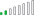cancel
Showing results for
Did you mean:New Member

## Syntax error

Hi, why this VAR - RETURN function is giving me error? It is correct until the first part "VAR_AVG_TL". The second formula is identical except for the standard deviation instead of the average. Thanks in advance

This the formula:

VAR _Avg_TL =
CALCULATE(
AVERAGE('Merge RPE duration'[TL]),
DATESINPERIOD('Merge RPE duration'[Date],LASTDATE('Merge RPE duration'[Date]),-7,DAY)
)
VAR _SD_TL =
CALCULATE(
STDEV.P('Merge RPE duration'[TL]),
DATESINPERIOD('Merge RPE duration'[Date],LASTDATE('Merge RPE duration'[Date]),-7,DAY)

VAR _Monotony = DIVIDE(_Avg_TL, _SD_TL)

RETURN
_Monotony

This is the error:
The syntax for 'VAR' is incorrect. (DAX(CALCULATE( AVERAGE('Merge RPE duration'[TL]), DATESINPERIOD('Merge RPE duration'[Date],LASTDATE('Merge RPE duration'[Date]),-7,DAY))VAR _SD_TL = CALCULATE( STDEV.P('Merge RPE duration'[TL]), DATESINPERIOD('Merge RPE duration'[Date],LASTDATE('Merge RPE duration'[Date]),-7,DAY)VAR _Monotony = DIVIDE(_Avg_TL, _SD_TL)RETURN _Monotony)).
3 REPLIES 3Resolver II

Hi,

Here is the edited version of measure.

Best Regards,

Ezgi Naz Aslankara

If this post helps, then please consider Accept it as the solution to help the other members find it more quickly

measure = VAR _Avg_TL=
CALCULATE(
AVERAGE('Merge RPE duration'[TL]),
DATESINPERIOD('Merge RPE duration'[Date],LASTDATE('Merge RPE duration'[Date]),-7,DAY)
)
VAR _SD_TL =
CALCULATE(
STDEV.P('Merge RPE duration'[TL]),
DATESINPERIOD('Merge RPE duration'[Date],LASTDATE('Merge RPE duration'[Date]),-7,DAY))

VAR _Monotony = DIVIDE(_Avg_TL , _SD_TL)

RETURN
_MonotonySolution Sage

Hello,

Try this one

VAR _Avg_TL =
CALCULATE(
AVERAGE('Merge RPE duration'[TL]),
DATESINPERIOD('Merge RPE duration'[Date],LASTDATE('Merge RPE duration'[Date]),-7,DAY)
)
VAR _SD_TL =
CALCULATE(
STDEV.P('Merge RPE duration'[TL]),
DATESINPERIOD('Merge RPE duration'[Date],LASTDATE('Merge RPE duration'[Date]),-7,DAY))

VAR _Monotony = DIVIDE(_Avg_TL, _SD_TL)

RETURN
_MonotonySolution Sage

missing ")" before "VAR _Monotony..."